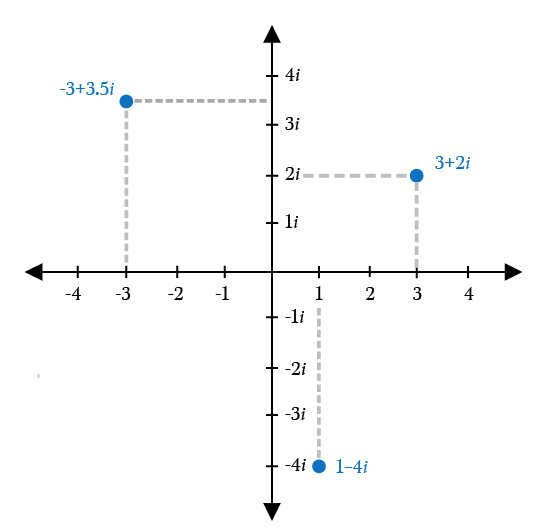or# RD Sharma Class 11 and Chapter Complex Numbers Maths Solutions

## Maths Class 11 and Chapter Complex Numbers RD Sharma SolutionsFilter
Filters :
CLASSES
•
•
•
•
•
•
•
CHAPTERS
•
• 23 More

## Complex Numbers: Class 11 Maths RD Sharma Solutions

### Complex Numbers :

These the numbers represented a + bi; where a and b are real numbers (including 0) and i is an imaginary number. Therefore a complex number contains two 'parts': one that is real and another part that is imaginary. Note that even though the complex number has an imaginary part, there are actually many real-world scientific applications of these "imaginary" number part such as oscillating springs and electronics.

### Plotting complex numbers:

Using the complex plane, you can plot complex numbers similar to how we plot a coordinate on the Cartesian plane. For example 3 + 2i, 1 – 4i and -3 + 3.5i All you need to do is draw a point at the intersection of the real part, found on the horizontal axis, and the imaginary part, found on the vertical axis. Likewise, you will be learning Adding & Subtracting Complex Numbers, Multiplying Complex Numbers, Dividing Complex Numbers, The Complex Conjugate. Every topic is explained in detailed using graphical illustrations. Practice given RD Sharma exercises questions for the chapter 13 Complex Numbers now!

### Topics and Subtopics of RD Sharma Class 11 Solutions Chapter 13 Complex Numbers

In RD Sharma Class 11 Solutions Chapter 13 Complex Numbers you will come across different operations on complex numbers including addition, subtraction, multiplication, division, conjugate, modulus and reciprocal of complex numbers. Find the answer to all the questions in the Chapter 13 Complex Numbers R.D. Sharma Class 11 Maths textbook. The solutions are designed according to the latest CBSE syllabus. The detailed RD Sharma Class 11 Solutions - Chapter 13 Complex Numbers will help you build a strong foundation of the basic Mathematics concepts that can help you ace the school level exam as well as other competitive exams. Exercise-Wise solutions include:

1. Detailed illustration of solving each problem.

2. Clarification of all the concepts and formulae used.

3. Use of algorithmic techniques to reach the solution.

Explained here are Topics and Subtopics of RD Sharma Class 11 Solutions Chapter 13 Complex Numbers. It will help you to go deeper into the number system and aid you to find alternate outlooks for resolving a problem. Some of the questions are simply formula based and are quite easy to crack while others may demand tricks. Thus provided solutions are a perfect blend of questions of all levels.

 Chapter 13  Complex Numbers Complex Numbers Exercise 13.1 Complex Numbers Exercise 13.2 Complex Numbers Exercise 13.3 Complex Numbers Exercise 13.4

To learn more, click on the exercise link given above for the RD Sharma Class 11 Solutions - Chapter 13 Complex Numbers comprising study notes, video lectures, previous year solved questions, etc.